# Balance Equation v004

14 de Mar de 2013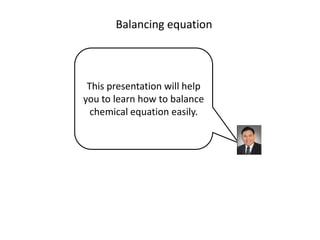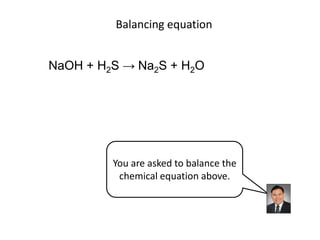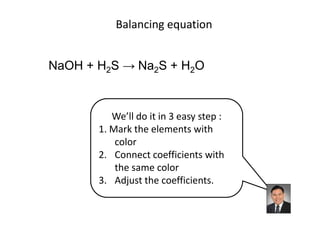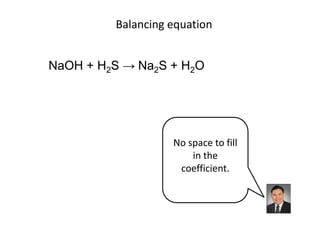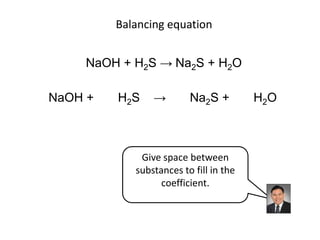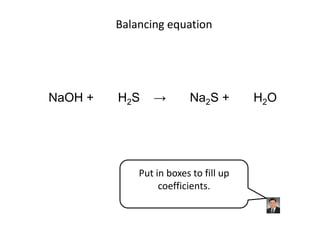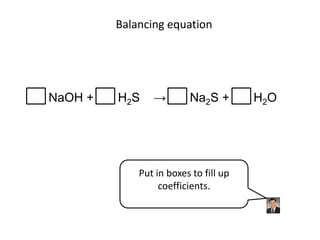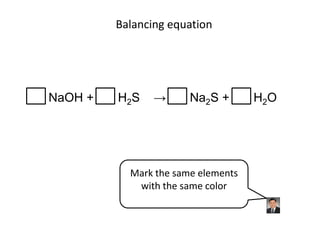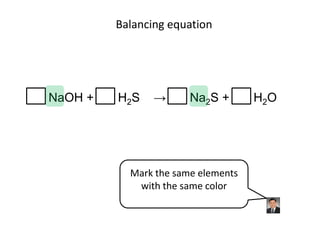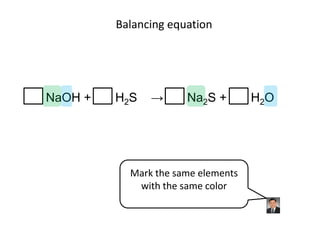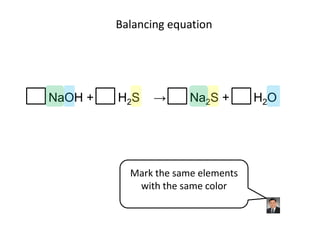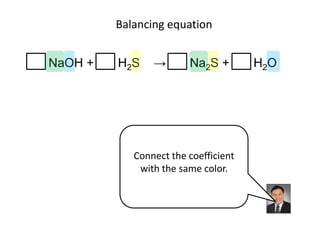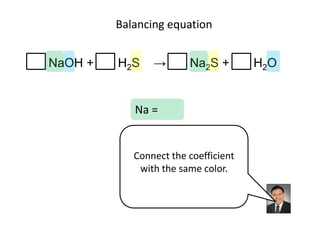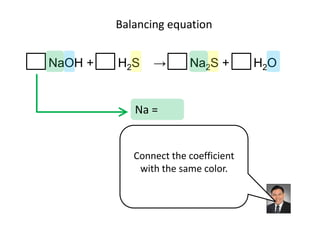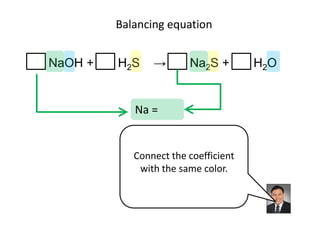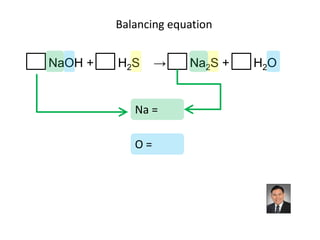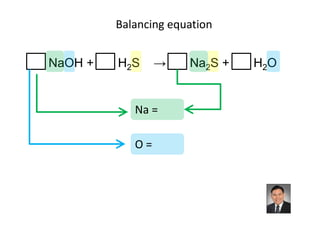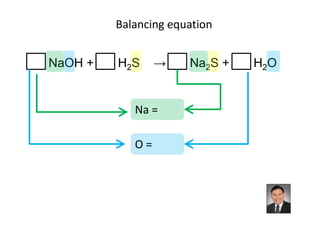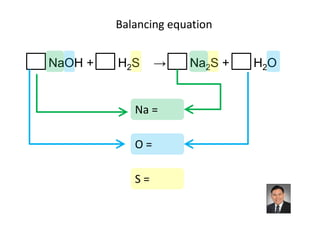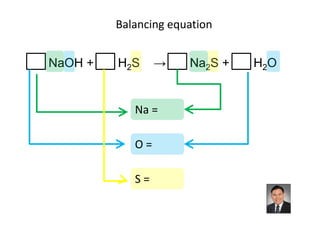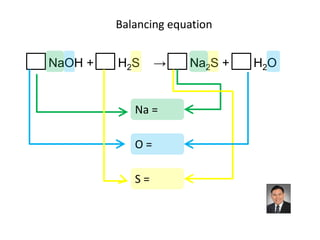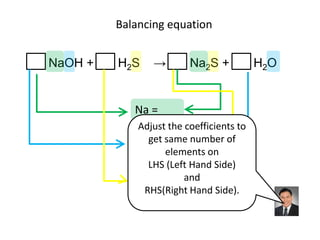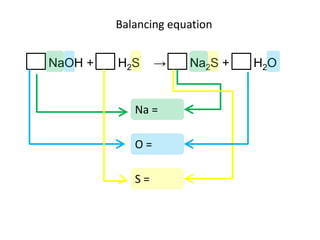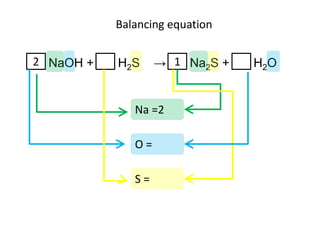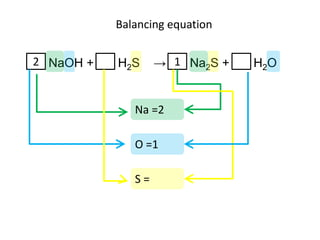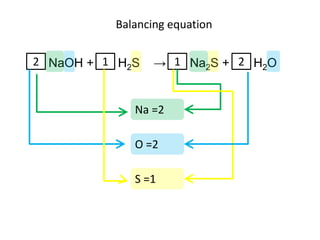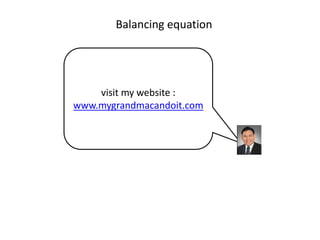1 de 27

### Notas del editor

1. This presentation will help you to learn how to balance chemical equation easily.
2. You are asked to balance the chemical equation above.
4. There is not enough space to fill up the coefficient.
5. Give space between substances to fill in the coefficient.
6. That’s it.
7. Mark the same elements with the same color
8. Connect the coefficient with the same color.
9. to balance sodium.
10. Connect N A O H
11. and N A two S
12. To balance oxygen
13. Connect N A O H.
14. And H two O.
15. To balance sulfur.
16. Connect H two S.
17. And N A two S.
18. Adjust the coefficients to get same number of elements onLeft Hand Side and Right Hand Side.
19. There is one N A on the left and two N A on the right.